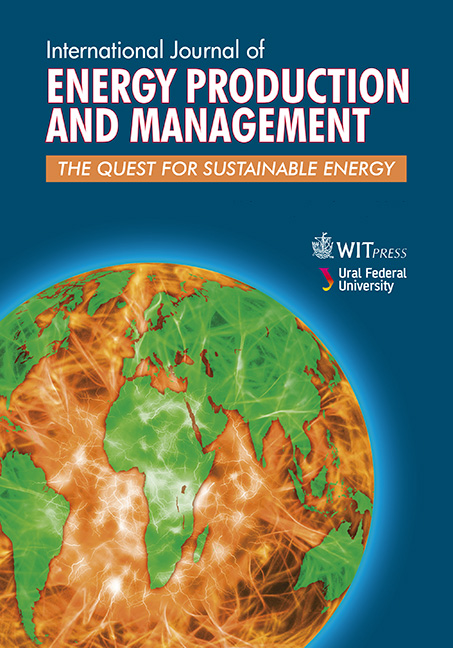WIT Press

Energy increase by use of a 2-parameter rule curve for hydropower generation

Price

Free (open access)Volume

Volume 2 (2017), Issue 1

Pages

13

Page Range

81 - 94

Paper DOI

10.2495/EQ-V2-N1-81-94

WIT Press

Author(s)

HORACIO L. VELASCO

Abstract

A new 2-Parameter Rule Curve (patent pending) for Hydropower Generation takes into account the current state of the system, represented by the beginning-of-month reservoir level, in the selection of the end-of-month Rule Curve level/storage. Thus, the two decision parameters are current time and reservoir level. The Rule Curve aims to preserve the firm energy, and to generate as much secondary energy as possible; and is based on the maximum monthly value of a composite parameter related to the variation of the potential energy stored in the reservoir, calculated for every month in the backward simulation that gives the Firm Energy Yield of the reservoir-power plant system. The Rule Curve levels are calculated to give the required end-of-month level for each month as a function of the beginning-of-month level, can be represented in tabular (matrix) and graphical forms, and can be used alone or combined with the Rule Curve proposed by the USACE (1985 and 1989), whose only parameter is current time. The use of this new 2-Parameter Rule Curve for single reservoirs can increase the annual average/secondary energy output without reducing the firm energy, as tested by the simulation of several hydropower developments; the results show an increase of average/secondary energy when compared with the USACE Rule Curve. Energy increase depends on net head variability, regulating capacity of the reservoir and power-plant-rated capacity. A 50-year simulation of Grand Coulee Dam gives an average energy output of 22.6 TW-h/year with the 2-Parameter Rule Curve, and 20.9 TW-h/ year with the USACE Rule Curve. The increase in average/secondary energy is 1.7 TW-h/year, due only to the change of the Rule Curve. Data published by the USBR indicates an average output of 21 TW-h/year for Grand Coulee Dam, which confirms the simulated results.

Keywords

hydropower, potential energy, reservoirs, rule curve, simulation